Function Repository Resource:

# PointArrayToPolygons

Make polygons from an array of points

Contributed by: Jan Mangaldan
 ResourceFunction["PointArrayToPolygons"][array] returns a GraphicsComplex object representing polygons formed from the two-dimensional array of 3D points array.

## Details and Options

The points in ResourceFunction["PointArrayToPolygons"] can also take the form {x,y,z,col}, where col is a color directive.
ResourceFunction["PointArrayToPolygons"] takes the option VertexNormals. With , vertex normals are automatically computed.

## Examples

### Basic Examples (2)

An array of points:

 In:=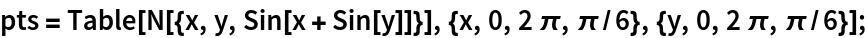Make polygons out of the points, and visualize them:

 In:=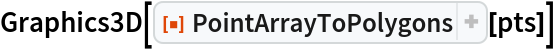Out=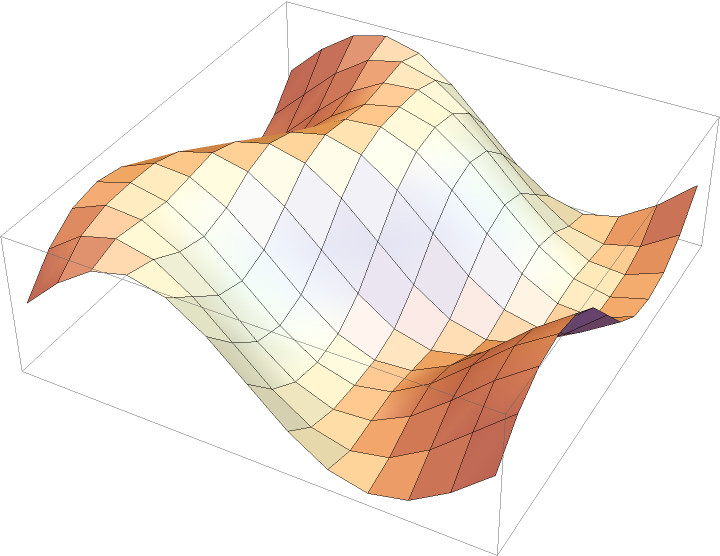### Scope (2)

Points representing a torus:

 In:=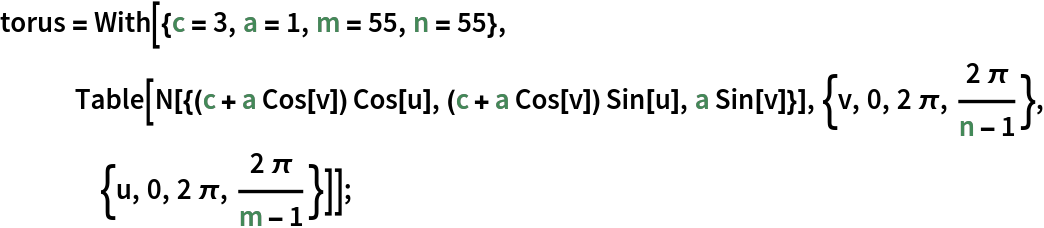Use directives to modify the style of the polygons:

 In:=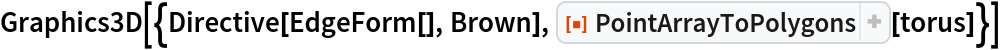Out=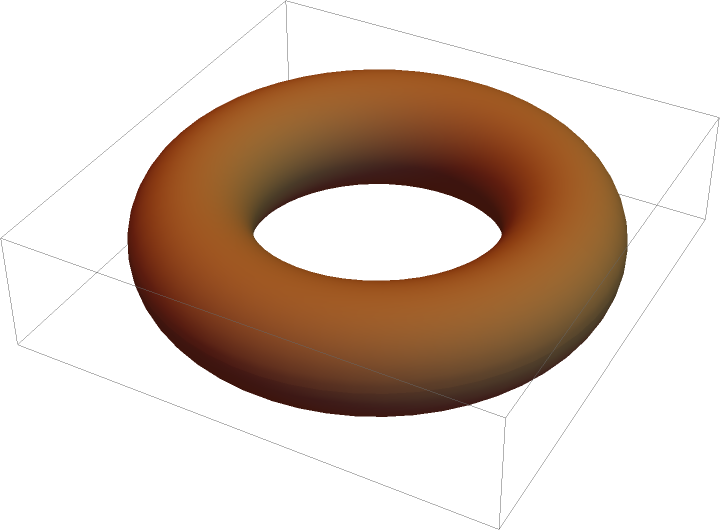Torus points with color directives for each point:

 In:=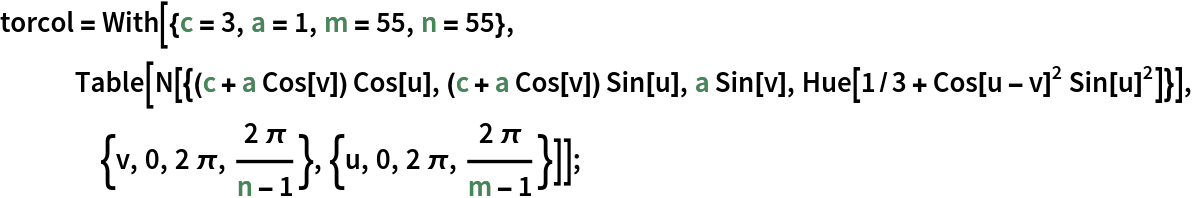Show the colored torus:

 In:=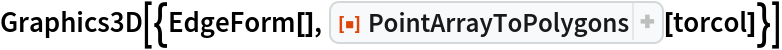Out=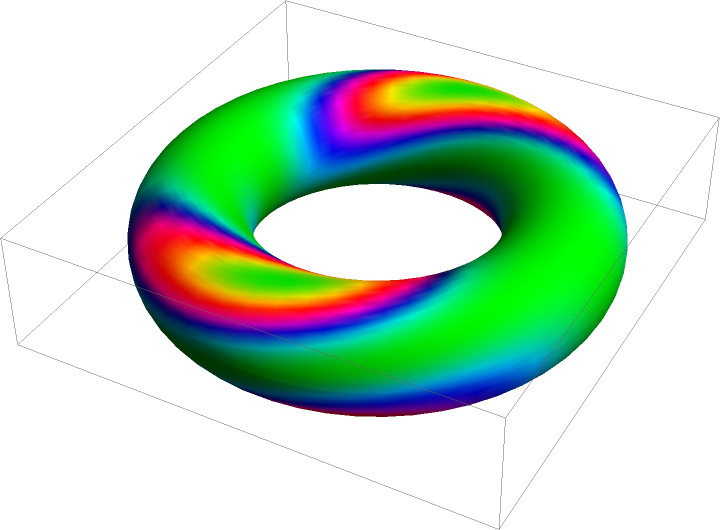### Options (1)

#### VertexNormals (1)

Compare the result of including and not including normals:

 In:=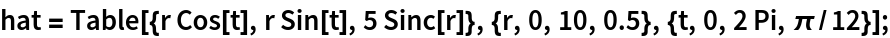In:=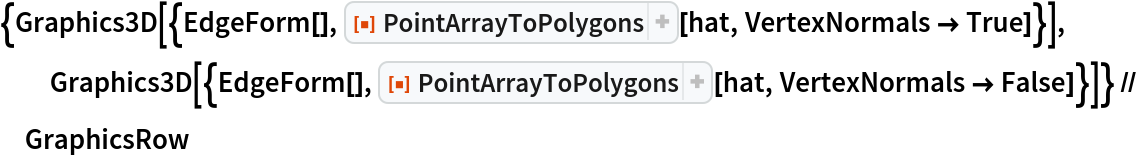Out=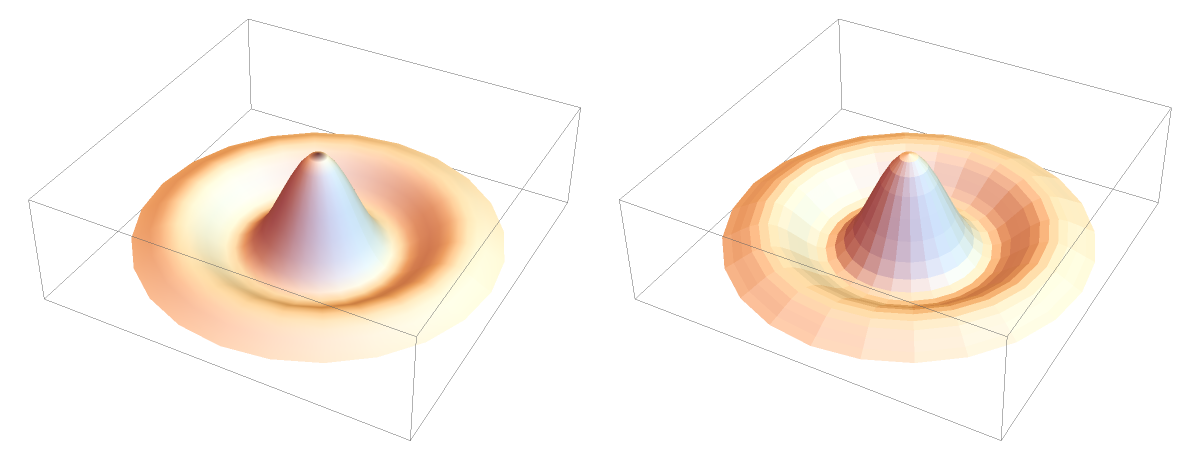### Neat Examples (1)

Use PointArrayToPolygons with the resource function PerforatePolygons to make a surface with holes:

 In:=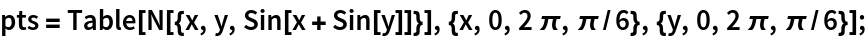In:=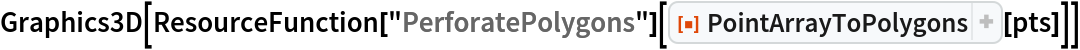Out=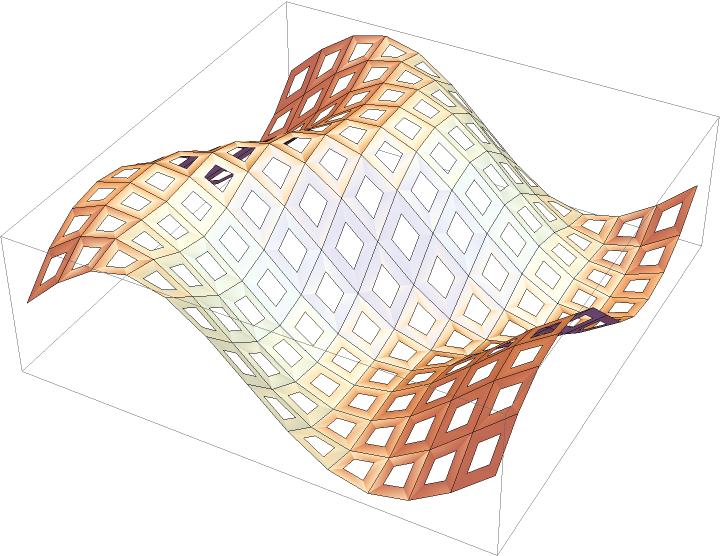## Version History

• 1.0.0 – 04 February 2021

## Author Notes

This is based on Roman Maeder's MakePolygons function in "Programming in Mathematica".